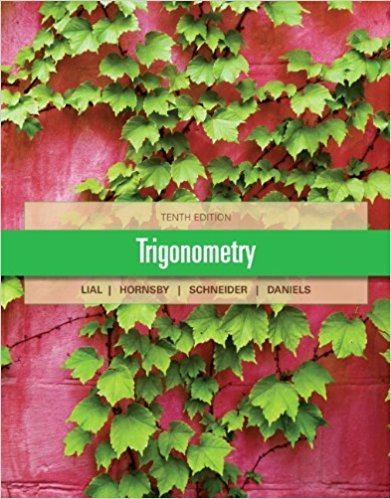×
×

# Solutions for Chapter 6: Review Exercises## Full solutions for Trigonometry | 10th Edition

ISBN: 9780321671776Solutions for Chapter 6: Review Exercises

Solutions for Chapter 6
4 5 0 304 Reviews
25
1
##### ISBN: 9780321671776

This textbook survival guide was created for the textbook: Trigonometry, edition: 10. Since 68 problems in chapter 6: Review Exercises have been answered, more than 35429 students have viewed full step-by-step solutions from this chapter. This expansive textbook survival guide covers the following chapters and their solutions. Trigonometry was written by and is associated to the ISBN: 9780321671776. Chapter 6: Review Exercises includes 68 full step-by-step solutions.

Key Math Terms and definitions covered in this textbook
• Characteristic equation det(A - AI) = O.

The n roots are the eigenvalues of A.

• Condition number

cond(A) = c(A) = IIAIlIIA-III = amaxlamin. In Ax = b, the relative change Ilox III Ilx II is less than cond(A) times the relative change Ilob III lib II· Condition numbers measure the sensitivity of the output to change in the input.

• Cramer's Rule for Ax = b.

B j has b replacing column j of A; x j = det B j I det A

• Diagonalization

A = S-1 AS. A = eigenvalue matrix and S = eigenvector matrix of A. A must have n independent eigenvectors to make S invertible. All Ak = SA k S-I.

• Distributive Law

A(B + C) = AB + AC. Add then multiply, or mUltiply then add.

• Identity matrix I (or In).

Diagonal entries = 1, off-diagonal entries = 0.

• Jordan form 1 = M- 1 AM.

If A has s independent eigenvectors, its "generalized" eigenvector matrix M gives 1 = diag(lt, ... , 1s). The block his Akh +Nk where Nk has 1 's on diagonall. Each block has one eigenvalue Ak and one eigenvector.

• Linearly dependent VI, ... , Vn.

A combination other than all Ci = 0 gives L Ci Vi = O.

• Minimal polynomial of A.

The lowest degree polynomial with meA) = zero matrix. This is peA) = det(A - AI) if no eigenvalues are repeated; always meA) divides peA).

• Network.

A directed graph that has constants Cl, ... , Cm associated with the edges.

• Normal equation AT Ax = ATb.

Gives the least squares solution to Ax = b if A has full rank n (independent columns). The equation says that (columns of A)·(b - Ax) = o.

• Particular solution x p.

Any solution to Ax = b; often x p has free variables = o.

• Random matrix rand(n) or randn(n).

MATLAB creates a matrix with random entries, uniformly distributed on [0 1] for rand and standard normal distribution for randn.

• Rank r (A)

= number of pivots = dimension of column space = dimension of row space.

• Rayleigh quotient q (x) = X T Ax I x T x for symmetric A: Amin < q (x) < Amax.

Those extremes are reached at the eigenvectors x for Amin(A) and Amax(A).

• Solvable system Ax = b.

The right side b is in the column space of A.

• Symmetric matrix A.

The transpose is AT = A, and aU = a ji. A-I is also symmetric.

• Transpose matrix AT.

Entries AL = Ajj. AT is n by In, AT A is square, symmetric, positive semidefinite. The transposes of AB and A-I are BT AT and (AT)-I.

• Vector space V.

Set of vectors such that all combinations cv + d w remain within V. Eight required rules are given in Section 3.1 for scalars c, d and vectors v, w.

• Volume of box.

The rows (or the columns) of A generate a box with volume I det(A) I.

×